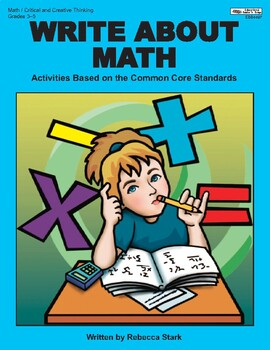# Write About Math: Activities Based on the Common Core Standards, Grades 3–5Subject
Resource Type
File Type

PDF

(6 MB|118 pages)
Product Description

Included are 100 activities based upon mathematics common core standards. Also included are 20 activities based upon math topics that encourage critical and creative thinking. All activities develop important writing skills as well. Pages include ample room for writing.

Click here to browse all math products by Rebecca Stark (Educational Books 'n' Bingo).

The lessons and activities in this book align with the following Common Core State Standards*:

CCSS.Math.Content.3.OA.A,B,C,D; NBT.A; NF.A; MD.A,B,C,D; G

CCSS.Math.Content.4.OA.A,B,C; NBT.A,B; NF.A,B,C; MD.A,B,C; G

CCSS.Math.Content.5.OA.A; NBT.A,B; NF.A,B; MD.A,B,C; G

OPERATIONS & ALGEBRAIC THINKING

Represent and solve problems involving multiplication and division.

Understand properties of multiplication and the relationship between multiplication and division.

Multiply and divide within 100.

Solve problems involving the four operations, and identify and explain patterns in arithmetic.

Use the four operations with whole numbers to solve problems.

Gain familiarity with factors and multiples.

Generate and analyze patterns.

Write and interpret numerical expressions.

Analyze patterns and relationships.

NUMBERS & OPERATIONS IN BASE 10

Use place value understanding and properties of operations to perform multi-digit arithmetic.

Generalize place value understanding for multi-digit whole numbers.

Use place value understanding and properties of operations to perform multi-digit arithmetic.

Understand the place value system.

Perform operations with multi-digit whole numbers and with decimals to hundredths.

NUMBERS & OPERATIONS —FRACTIONS

Develop understanding of fractions as numbers.

Extend understanding of fraction equivalence and ordering.

Build fractions from unit fractions.

Understand decimal notation for fractions, and compare decimal fractions.

Use equivalent fractions as a strategy to add and subtract fractions.

Apply and extend previous understandings of multiplication and division.

MEASUREMENT & DATA

Solve problems involving measurement and estimation.

Represent and interpret data.

Geometric measurement: understand concepts of area and relate area to multiplication and to addition.

Solve problems involving measurement and conversion of measurements.

Geometric measurement: understand concepts of angle and measure angles.

Convert like measurement units within a given measurement system.

Geometric measurement: understand concepts of volume.

GEOMETRY

Reason with shapes and their attributes.

Classify two-dimensional figures into categories based on their properties.

Total Pages
118 pages
Included
Teaching Duration
N/A
Report this Resource to TpT
Reported resources will be reviewed by our team. Report this resource to let us know if this resource violates TpT’s content guidelines.